# SilkBase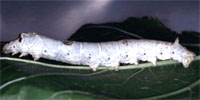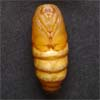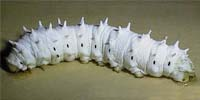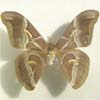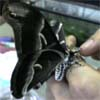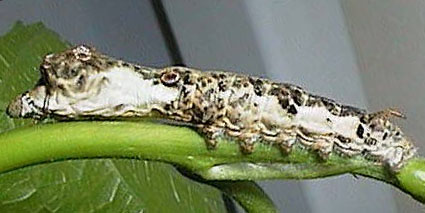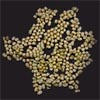Last updated: 2019/10/06

```BLASTX 2.2.12 [Aug-07-2005]

Reference: Altschul, Stephen F., Thomas L. Madden, Alejandro A. Schaffer,
Jinghui Zhang, Zheng Zhang, Webb Miller, and David J. Lipman (1997),
"Gapped BLAST and PSI-BLAST: a new generation of protein database search
programs",  Nucleic Acids Res. 25:3389-3402.

Query= tesS0007
(618 letters)

Database: nematostella
59,808 sequences; 16,821,457 total letters

Searching..................................................done

Score    E
Sequences producing significant alignments:                      (bits) Value

SB_47515| Best HMM Match : zf-C2H2 (HMM E-Value=0.13)                  29   2.3
SB_17452| Best HMM Match : zf-C2H2 (HMM E-Value=0.13)                  29   2.3
SB_45345| Best HMM Match : No HMM Matches (HMM E-Value=.)              28   5.3
SB_54554| Best HMM Match : zf-C2H2 (HMM E-Value=0.13)                  28   7.0
SB_52808| Best HMM Match : zf-C2H2 (HMM E-Value=0.13)                  28   7.0
SB_41510| Best HMM Match : Minor_tail_Z (HMM E-Value=3.5)              28   7.0
SB_40055| Best HMM Match : zf-C2H2 (HMM E-Value=0.13)                  28   7.0
SB_36653| Best HMM Match : ACBP (HMM E-Value=3.6)                      28   7.0
SB_25596| Best HMM Match : No HMM Matches (HMM E-Value=.)              28   7.0
SB_20592| Best HMM Match : No HMM Matches (HMM E-Value=.)              28   7.0
SB_14108| Best HMM Match : zf-C2H2 (HMM E-Value=0.13)                  28   7.0
SB_9519| Best HMM Match : zf-C2H2 (HMM E-Value=0.13)                   28   7.0
SB_5126| Best HMM Match : No HMM Matches (HMM E-Value=.)               28   7.0
SB_3604| Best HMM Match : No HMM Matches (HMM E-Value=.)               28   7.0
SB_2129| Best HMM Match : No HMM Matches (HMM E-Value=.)               28   7.0
SB_54746| Best HMM Match : RVT_1 (HMM E-Value=8.4e-11)                 28   7.0
SB_36062| Best HMM Match : zf-C2H2 (HMM E-Value=0.13)                  28   7.0
SB_32612| Best HMM Match : zf-C2H2 (HMM E-Value=0.13)                  28   7.0
SB_5327| Best HMM Match : zf-C2H2 (HMM E-Value=0.13)                   28   7.0
SB_36229| Best HMM Match : zf-C2H2 (HMM E-Value=0.13)                  27   9.2

>SB_47515| Best HMM Match : zf-C2H2 (HMM E-Value=0.13)
Length = 189

Score = 29.5 bits (63), Expect = 2.3
Identities = 27/94 (28%), Positives = 40/94 (42%), Gaps = 2/94 (2%)
Frame = +1

DS   E       ++ W DR +   +L +  L      + K +T W   A HV   +D
Sbjct: 15  DSSFMEDAILNILNIRWQDRITNTEVLERANLPSVITTMRKAQTRW---AGHVCRMSDSR 71

Query: 499 CKKSEI*GELILGS*KRIG*AKKSNGCL*LHVKE 600
K  + GEL  GS K  G  K+   C+  H+K+
Sbjct: 72  IPKQLLYGELSRGSRKVGGQRKRYKDCIKSHLKD 105

>SB_17452| Best HMM Match : zf-C2H2 (HMM E-Value=0.13)
Length = 189

Score = 29.5 bits (63), Expect = 2.3
Identities = 27/94 (28%), Positives = 40/94 (42%), Gaps = 2/94 (2%)
Frame = +1

DS   E       ++ W DR +   +L +  L      + K +T W   A HV   +D
Sbjct: 15  DSSFMEDAILNILNIRWQDRITNTEVLERANLPSVITTMRKAQTRW---AGHVCRMSDSR 71

Query: 499 CKKSEI*GELILGS*KRIG*AKKSNGCL*LHVKE 600
K  + GEL  GS K  G  K+   C+  H+K+
Sbjct: 72  IPKQLLYGELSRGSRKVGGQRKRYKDCIKSHLKD 105

>SB_45345| Best HMM Match : No HMM Matches (HMM E-Value=.)
Length = 2346

Score = 28.3 bits (60), Expect = 5.3
Identities = 13/27 (48%), Positives = 14/27 (51%)
Frame = +1

Query: 262  ETGENRQFIHINEPPIIVQEHDSQPQE 342
E GE  Q  HIN   I+V E    PQE
Sbjct: 1139 EVGEELQIFHINPGSIVVTELPPSPQE 1165

>SB_54554| Best HMM Match : zf-C2H2 (HMM E-Value=0.13)
Length = 137

Score = 27.9 bits (59), Expect = 7.0
Identities = 19/56 (33%), Positives = 27/56 (48%)
Frame = +1

+ K +T W   A HV   +D    K  + GEL  GS K  G  K+   C+  H+K+
Sbjct: 1   MRKAQTRW---AGHVCRMSDSRIPKQLLYGELSRGSRKVGGQRKRYKDCIKSHLKD 53

>SB_52808| Best HMM Match : zf-C2H2 (HMM E-Value=0.13)
Length = 166

Score = 27.9 bits (59), Expect = 7.0
Identities = 19/56 (33%), Positives = 27/56 (48%)
Frame = +1

+ K +T W   A HV   +D    K  + GEL  GS K  G  K+   C+  H+K+
Sbjct: 30  MRKAQTRW---AGHVCRMSDSRIPKQLLYGELSRGSRKVGGQRKRYKDCIKSHLKD 82

>SB_41510| Best HMM Match : Minor_tail_Z (HMM E-Value=3.5)
Length = 208

Score = 27.9 bits (59), Expect = 7.0
Identities = 19/56 (33%), Positives = 27/56 (48%)
Frame = +1

+ K +T W   A HV   +D    K  + GEL  GS K  G  K+   C+  H+K+
Sbjct: 141 MRKAQTRW---AGHVCRMSDSRIPKQLLYGELSRGSRKVGGQRKRYKDCIKSHLKD 193

>SB_40055| Best HMM Match : zf-C2H2 (HMM E-Value=0.13)
Length = 303

Score = 27.9 bits (59), Expect = 7.0
Identities = 19/56 (33%), Positives = 27/56 (48%)
Frame = +1

+ K +T W   A HV   +D    K  + GEL  GS K  G  K+   C+  H+K+
Sbjct: 167 MRKAQTRW---AGHVCRMSDSRIPKQLLYGELSRGSRKVGGQRKRYKDCIKSHLKD 219

>SB_36653| Best HMM Match : ACBP (HMM E-Value=3.6)
Length = 206

Score = 27.9 bits (59), Expect = 7.0
Identities = 10/28 (35%), Positives = 16/28 (57%)
Frame = +1

FK+ NK++  W  +A   G + DE  +K
Sbjct: 39  FKDKNKKENAWKQVAEQAGCSVDEAKRK 66

>SB_25596| Best HMM Match : No HMM Matches (HMM E-Value=.)
Length = 89

Score = 27.9 bits (59), Expect = 7.0
Identities = 10/28 (35%), Positives = 16/28 (57%)
Frame = +1

FK+ NK++  W  +A   G + DE  +K
Sbjct: 39  FKDKNKKENAWKQVAEQAGCSVDEAKRK 66

>SB_20592| Best HMM Match : No HMM Matches (HMM E-Value=.)
Length = 137

Score = 27.9 bits (59), Expect = 7.0
Identities = 19/56 (33%), Positives = 27/56 (48%)
Frame = +1

+ K +T W   A HV   +D    K  + GEL  GS K  G  K+   C+  H+K+
Sbjct: 1   MRKAQTRW---AGHVCRMSDSRIPKQLLYGELSRGSRKVGGQRKRYKDCIKSHLKD 53

>SB_14108| Best HMM Match : zf-C2H2 (HMM E-Value=0.13)
Length = 404

Score = 27.9 bits (59), Expect = 7.0
Identities = 19/56 (33%), Positives = 27/56 (48%)
Frame = +1

+ K +T W   A HV   +D    K  + GEL  GS K  G  K+   C+  H+K+
Sbjct: 268 MRKAQTRW---AGHVCRMSDSRIPKQLLYGELSRGSRKVGGQRKRYKDCIKSHLKD 320

>SB_9519| Best HMM Match : zf-C2H2 (HMM E-Value=0.13)
Length = 299

Score = 27.9 bits (59), Expect = 7.0
Identities = 19/56 (33%), Positives = 27/56 (48%)
Frame = +1

+ K +T W   A HV   +D    K  + GEL  GS K  G  K+   C+  H+K+
Sbjct: 163 MRKAQTRW---AGHVCRMSDSRIPKQLLYGELSRGSRKVGGQRKRYKDCIKSHLKD 215

>SB_5126| Best HMM Match : No HMM Matches (HMM E-Value=.)
Length = 358

Score = 27.9 bits (59), Expect = 7.0
Identities = 19/56 (33%), Positives = 27/56 (48%)
Frame = +1

+ K +T W   A HV   +D    K  + GEL  GS K  G  K+   C+  H+K+
Sbjct: 222 MRKAQTRW---AGHVCRMSDSRIPKQLLYGELSRGSRKVGGQRKRYKDCIKSHLKD 274

>SB_3604| Best HMM Match : No HMM Matches (HMM E-Value=.)
Length = 1319

Score = 27.9 bits (59), Expect = 7.0
Identities = 19/56 (33%), Positives = 27/56 (48%)
Frame = +1

+ K +T W   A HV   +D    K  + GEL  GS K  G  K+   C+  H+K+
Sbjct: 1183 MRKAQTRW---AGHVCRMSDSRIPKQLLYGELSRGSRKVGGQRKRYKDCIKSHLKD 1235

>SB_2129| Best HMM Match : No HMM Matches (HMM E-Value=.)
Length = 166

Score = 27.9 bits (59), Expect = 7.0
Identities = 19/56 (33%), Positives = 27/56 (48%)
Frame = +1

+ K +T W   A HV   +D    K  + GEL  GS K  G  K+   C+  H+K+
Sbjct: 30  MRKAQTRW---AGHVCRMSDSRIPKQLLYGELSRGSRKVGGQRKRYKDCIKSHLKD 82

>SB_54746| Best HMM Match : RVT_1 (HMM E-Value=8.4e-11)
Length = 959

Score = 27.9 bits (59), Expect = 7.0
Identities = 19/56 (33%), Positives = 27/56 (48%)
Frame = +1

+ K +T W   A HV   +D    K  + GEL  GS K  G  K+   C+  H+K+
Sbjct: 887  MRKAQTRW---AGHVCRMSDSRIPKQLLYGELSRGSRKVGGQRKRYKDCIKSHLKD 939

>SB_36062| Best HMM Match : zf-C2H2 (HMM E-Value=0.13)
Length = 166

Score = 27.9 bits (59), Expect = 7.0
Identities = 19/56 (33%), Positives = 27/56 (48%)
Frame = +1

+ K +T W   A HV   +D    K  + GEL  GS K  G  K+   C+  H+K+
Sbjct: 30  MRKAQTRW---AGHVCRMSDSRIPKQLLYGELSRGSRKVGGQRKRYKDCIKSHLKD 82

>SB_32612| Best HMM Match : zf-C2H2 (HMM E-Value=0.13)
Length = 166

Score = 27.9 bits (59), Expect = 7.0
Identities = 19/56 (33%), Positives = 27/56 (48%)
Frame = +1

+ K +T W   A HV   +D    K  + GEL  GS K  G  K+   C+  H+K+
Sbjct: 30  MRKAQTRW---AGHVCRMSDSRIPKQLLYGELSRGSRKVGGQRKRYKDCIKSHLKD 82

>SB_5327| Best HMM Match : zf-C2H2 (HMM E-Value=0.13)
Length = 328

Score = 27.9 bits (59), Expect = 7.0
Identities = 19/56 (33%), Positives = 27/56 (48%)
Frame = +1

+ K +T W   A HV   +D    K  + GEL  GS K  G  K+   C+  H+K+
Sbjct: 192 MRKAQTRW---AGHVCRMSDSRIPKQLLYGELSRGSRKVGGQRKRYKDCIKSHLKD 244

>SB_36229| Best HMM Match : zf-C2H2 (HMM E-Value=0.13)
Length = 269

Score = 27.5 bits (58), Expect = 9.2
Identities = 19/56 (33%), Positives = 27/56 (48%)
Frame = +1

+ K +T W   A HV   +D    K  + GEL  GS K  G  K+   C+  H+K+
Sbjct: 133 MRKVQTRW---AGHVCRMSDSRIPKQLLYGELSRGSRKVGGQRKRYKDCIKSHLKD 185

Database: nematostella
Posted date:  Oct 22, 2007  1:22 PM
Number of letters in database: 16,821,457
Number of sequences in database:  59,808

Lambda     K      H
0.318    0.134    0.401

Gapped
Lambda     K      H
0.279   0.0580    0.190

Matrix: BLOSUM62
Gap Penalties: Existence: 9, Extension: 2
Number of Hits to DB: 17,634,508
Number of Sequences: 59808
Number of extensions: 331078
Number of successful extensions: 801
Number of sequences better than 10.0: 20
Number of HSP's better than 10.0 without gapping: 738
Number of HSP's successfully gapped in prelim test: 0
Number of HSP's that attempted gapping in prelim test: 0
Number of HSP's gapped (non-prelim): 801
length of database: 16,821,457
effective HSP length: 79
effective length of database: 12,096,625
effective search space used: 1524174750
frameshift window, decay const: 40,  0.1
T: 12
A: 40
X1: 16 ( 7.3 bits)
X2: 37 (14.9 bits)
X3: 62 (25.0 bits)
S1: 41 (21.7 bits)
```

The construction of this database has been supported by
Grant-in-Aid for Publication of Scientific Research Results,
JSPS, Japan (1999-2003, 2005-2018).

The EST sequencing was supported by the Genome Analysis Program,National Bioresource Project (NBRP),
Grants-in-Aid for Scientific Research, MEXT,and the Agrigenome Program, NIAS/MAFF.
The maintenance and distribution of the DNA clones are supported by the National Bioresource Project (NBRP).